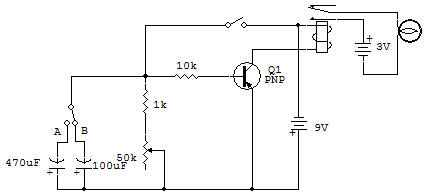Recherche personnalisé / personalised search

Transistor Delay Circuit Using RC Time ConstantThe purpose of this project is to demonstrate and study the effects of our RC (resistance, capacitance) time constant circuit.

This is accomplished by using the RC time constant circuit to control a switching Transistor which in turn controls the Relay which finally turns OFF the Lamp. The slide switch gives you a choice of two different Capacitors: 100uF or 470uF.

Consider operation with one of the Capacitors in the circuit and with the 50K Control set on maximum resistance (full CCW rotation or zero on the dial).

When the Key is closed the current through the 10K turns the Transistor full ON. This causes a virtual short circuit to appear between C-E of the 2SA, thereby energizing the Relay. The above turn OFF delay is dependent upon the product of R and C in the base circuit. The higher the product, the longer the delay. The smaller the product, the shorter the delay.

Because of the parallel paths of resistance, the effective base circuit resistance is calculated as adjustable between about 910 ohms and 8.4K ohm. It is this effective resistance that, when multiplied by the circuit capacitance, yields the time content of the circuit in seconds. This time gives us a relative indication of how long the delay will take.

For example, with the 100uF Capacitor the time constants at the R extremes are: 0.09 second and 0.8 second, for the 470uF: 0.4 second and 4 seconds.

Now if we consider that it takes about five times the constants for the voltage to reduce to near zero volts, the delay times are approximately 5 times the above time constant extremes. These calculate to be from about 0.45 second to 4 seconds for the 100uF Capacitor, and from about 2 seconds to 20 seconds for the 470uF Capacitor.

Now a measurement of the above extremes on the actual circuit may show differences in the times obtained because of component tolerances, but the relative values will be very close. Use a watch or clock with a second hand and check the results for yourself.

Recherche personnalisée